CLAT  >  Test: Data Interpretation- 1

# Test: Data Interpretation- 1 - CLAT

Test Description

## 30 Questions MCQ Test Quantitative Techniques for CLAT - Test: Data Interpretation- 1

Test: Data Interpretation- 1 for CLAT 2023 is part of Quantitative Techniques for CLAT preparation. The Test: Data Interpretation- 1 questions and answers have been prepared according to the CLAT exam syllabus.The Test: Data Interpretation- 1 MCQs are made for CLAT 2023 Exam. Find important definitions, questions, notes, meanings, examples, exercises, MCQs and online tests for Test: Data Interpretation- 1 below.
Solutions of Test: Data Interpretation- 1 questions in English are available as part of our Quantitative Techniques for CLAT for CLAT & Test: Data Interpretation- 1 solutions in Hindi for Quantitative Techniques for CLAT course. Download more important topics, notes, lectures and mock test series for CLAT Exam by signing up for free. Attempt Test: Data Interpretation- 1 | 30 questions in 45 minutes | Mock test for CLAT preparation | Free important questions MCQ to study Quantitative Techniques for CLAT for CLAT Exam | Download free PDF with solutions
 1 Crore+ students have signed up on EduRev. Have you?
Test: Data Interpretation- 1 - Question 1

### Study the following graph and answer the questions that follow. Number of Appeared Candidates and Passed Candidates (in hundreds) in a test from seven different Institutions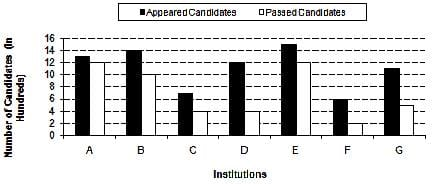Q. What is the difference between the number of candidates appeared from institutions B, C, D and F together and candidates passed from institutions A, E and G together?

Detailed Solution for Test: Data Interpretation- 1 - Question 1

Required difference:-

=> ( 14+7+12+6) -(12+12+5)

=>  39 - 29 = 10 hundred

=> 1000

Test: Data Interpretation- 1 - Question 2

### Study the following graph and answer the questions that follow. Number of Appeared Candidates and Passed Candidates (in hundreds) in a test from seven different InstitutionsQ. What is the average number of candidates passed from all the institutions together?

Test: Data Interpretation- 1 - Question 3

### Study the following graph and answer the questions that follow. Number of Appeared Candidates and Passed Candidates (in hundreds) in a test from seven different InstitutionsQ. The number of candidates passed from institutions C and E together is approximately what percentage of the total number of candidates appeared from institutions A and G together?

Test: Data Interpretation- 1 - Question 4

Study the following graph and answer the questions that follow.
Number of Appeared Candidates and Passed Candidates (in hundreds) in a test from seven different InstitutionsQ. From which institution is the difference between the appeared candidates and passed candidates the maximum?

Test: Data Interpretation- 1 - Question 5

Study the following graph and answer the questions that follow.
Number of Appeared Candidates and Passed Candidates (in hundreds) in a test from seven different InstitutionsQ. What is the ratio of the number of candidates who have failed Institution B to the number of candidates who have appeared from institution F?

Test: Data Interpretation- 1 - Question 6

Study the following table carefully to answer the questions that follow.
Number of flights (in hundreds) cancelled in five different sates during six different years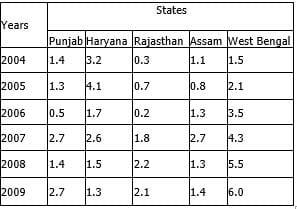Q. What was the respective ratio between the number of flights cancelled in Rajasthan in the year 2006 and number of flights cancelled in West Bengal in the year 2009?

Test: Data Interpretation- 1 - Question 7

Study the following table carefully to answer the questions that follow.
Number of flights (in hundreds) cancelled in five different sates during six different yearsQ. What was the average number of flights cancelled in all the state together in the year 2008?

Test: Data Interpretation- 1 - Question 8

Study the following table carefully to answer the questions that follow.
Number of flights (in hundreds) cancelled in five different sates during six different yearsQ. What is the difference between the number of flights cancelled in Assam in the year 2007 and the total number of flights cancelled din Rajasthan in the year 2005, 2007 and 2008 together?

Test: Data Interpretation- 1 - Question 9

Study the following table carefully to answer the questions that follow.
Number of flights (in hundreds) cancelled in five different sates during six different yearsQ. In which state the number of flights cancelled continuously increased during the years 2004 to 2009?

Test: Data Interpretation- 1 - Question 10

Study the following table carefully to answer the questions that follow.
Number of flights (in hundreds) cancelled in five different sates during six different yearsQ. Number of flights cancelled in Haryana in the year 2004 was what percent of the total number of flights  cancelled in Punjab over all the years together?

Test: Data Interpretation- 1 - Question 11

Study the following graph carefully and answer the question.
Water level of four major Rivers (in metres) in four different Months
Water level of four Rivers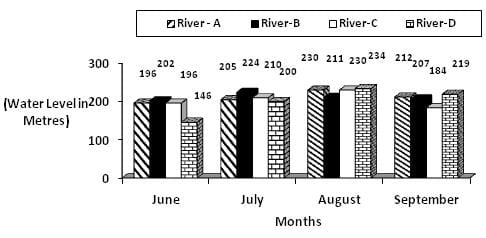Q. What was the respective ratio between the water level of River – C in September and the water level of River – B in June?

Test: Data Interpretation- 1 - Question 12

Study the following graph carefully and answer the question.
Water level of four major Rivers (in metres) in four different Months
Water level of four RiversQ. If the danger level of all the four rivers is above 215 m, which river has not crossed the danger level in August   but has crossed the danger level in July?

Test: Data Interpretation- 1 - Question 13

Study the following graph carefully and answer the question.
Water level of four major Rivers (in metres) in four different Months
Water level of four RiversQ. What is the average water level of River –A in all the four months together?

Detailed Solution for Test: Data Interpretation- 1 - Question 13

The correct answer is C, average water level of river A = (196+205+230+212)/4 = 210.75 m,

Test: Data Interpretation- 1 - Question 14

Study the following graph carefully and answer the question.
Water level of four major Rivers (in metres) in four different Months
Water level of four RiversQ. In which river and in which month respectively the water level is highest?

Test: Data Interpretation- 1 - Question 15

Study the following graph carefully and answer the question.
Water level of four major Rivers (in metres) in four different Months
Water level of four RiversQ. If the water level of River-A in July is decreased by 20%, then what will be the water level of River-A in July

Test: Data Interpretation- 1 - Question 16

Study the following table carefully to answer the questions that follow:
Number of Students Appeared (A) and Failed (F) in Five
Classes of a school over the years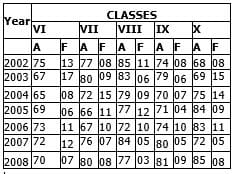Q. What is the number of passed students for all the classes together in the year 2007?

Detailed Solution for Test: Data Interpretation- 1 - Question 16

The correct answer is C as the calculation is shown below
VI=72-12=60
VII=76-7=69
VIII=84-5=79
IX=80-5=75
X=72-5=67
TOTAL = 60+69+79+75+67=350

Test: Data Interpretation- 1 - Question 17

Study the following table carefully to answer the questions that follow:
Number of Students Appeared (A) and Failed (F) in Five
Classes of a school over the years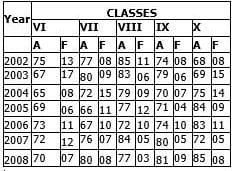Q. What is the average number of failed students from Class VIII for the given years?

Test: Data Interpretation- 1 - Question 18

Study the following table carefully to answer the questions that follow:
Number of Students Appeared (A) and Failed (F) in Five
Classes of a school over the yearsQ. What is the ratio of the total number of passed students to total number of failed students for the year 2005?

Test: Data Interpretation- 1 - Question 19

Study the following table carefully to answer the questions that follow:
Number of Students Appeared (A) and Failed (F) in Five
Classes of a school over the yearsQ. What is the total percentage of passed students of Class IX from all the years together? (rounded off to two digits after decimal)

Test: Data Interpretation- 1 - Question 20

Study the following table carefully to answer the questions that follow:
Number of Students Appeared (A) and Failed (F) in Five
Classes of a school over the yearsQ.
Which of the following classes has the minimum number of passed students over the years?

Test: Data Interpretation- 1 - Question 21

Study the following table carefully and answer the questions given follow:
Monthly expenditure (in thousands) by five people on Rent, Food, Children’s education, Clothes and Traveling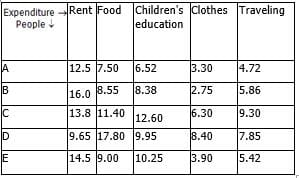Q. What is the total monthly expenditure made by D on rent, B on clothes and E on traveling together?

Test: Data Interpretation- 1 - Question 22

Study the following table carefully and answer the questions given follow:
Monthly expenditure (in thousands) by five people on Rent, Food, Children’s education, Clothes and TravelingQ. What is the average monthly expenditure on food by all the people together?

Test: Data Interpretation- 1 - Question 23

Study the following table carefully and answer the questions given follow:
Monthly expenditure (in thousands) by five people on Rent, Food, Children’s education, Clothes and TravelingQ. Whose monthly expenditure on all the heads together is the lowest among them?

Test: Data Interpretation- 1 - Question 24

Study the following table carefully and answer the questions given follow:
Monthly expenditure (in thousands) by five people on Rent, Food, Children’s education, Clothes and TravelingQ. If the monthly expenditure of C on Children’s education is increased by 5%, then what will be his yearly  expenditure on Children’s education?

Test: Data Interpretation- 1 - Question 25

Study the following table carefully and answer the questions given follow:
Monthly expenditure (in thousands) by five people on Rent, Food, Children’s education, Clothes and TravelingQ. What is the ratio of the monthly expenditure made by A on traveling to the monthly expenditure made by D on clothes?

Test: Data Interpretation- 1 - Question 26

Study the graph and answer the questions that follow:
No. of candidates (in thousands) different Qualified in the written test for admission to two different institutions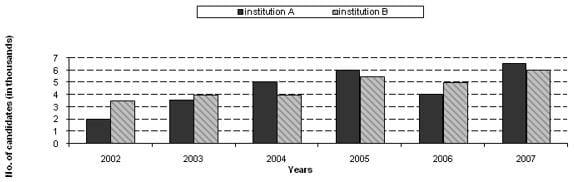Q. What was the ratio of the number of candidates qualified in the written test in the year 2002 for admission to  institution B to the number of candidates qualified in the written test in the year 2006 for admission to  institution A?

Test: Data Interpretation- 1 - Question 27

Study the graph and answer the questions that follow:
No. of candidates (in thousands) different Qualified in the written test for admission to two different institutionsQ. What was the approximate average number of candidates qualified in the written test for admission to  institution B over all the years?

Test: Data Interpretation- 1 - Question 28

Study the graph and answer the questions that follow:
No. of candidates (in thousands) different Qualified in the written test for admission to two different institutionsQ. In which year was the total number of candidates qualified in the written test for admission to both the institutions together the second highest?

Test: Data Interpretation- 1 - Question 29

Study the graph and answer the questions that follow:
No. of candidates (in thousands) different Qualified in the written test for admission to two different institutionsWhat is the difference between the total number of candidates qualified in written test in year 2006 for admission to institution A and B together and the number of candidates qualified in written test in year 2003 for admission to institution A ?

Detailed Solution for Test: Data Interpretation- 1 - Question 29

Required difference

= [(4+5) - 3.5] thousand

= 5500

Test: Data Interpretation- 1 - Question 30

Study the graph and answer the questions that follow:
No. of candidates (in thousands) different Qualified in the written test for admission to two different institutionsQ. What was the total number of candidates qualified in the written test for admission to institution A over all the years together?

## Quantitative Techniques for CLAT

46 videos|69 docs|95 tests
Information about Test: Data Interpretation- 1 Page
In this test you can find the Exam questions for Test: Data Interpretation- 1 solved & explained in the simplest way possible. Besides giving Questions and answers for Test: Data Interpretation- 1, EduRev gives you an ample number of Online tests for practice

## Quantitative Techniques for CLAT

46 videos|69 docs|95 tests